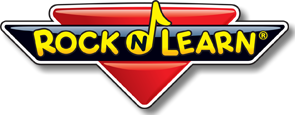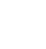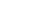﻿ Free Math WorksheetsFree Math Worksheets
from the creators of Rock 'N LearnOur worksheets for math go right along with Rock ’N Learn math audio CDs and math DVDs.

You do not have to purchase Rock ’N Learn programs to use these worksheets, but they certainly compliment one another.

Click a quick link below to get started

Rap Themed

A-maze-ing Facts (AP04)

Addends up to 4 – Maze

Sum Colors (AP10)

Addends up to 9 – Color Fill

Addends up to 9 – Dot-to-Dot

Maze Craze! (AP21)

Addends up to 9 – Maze

Search, Find, Circle (AP22)

Mixed Addition – Seek & Find

Subtraction

Rap Themed

A-maze-ing Math (SP04)

Subtract from up to 5 – Maze

See the Difference! (SP09)

From up to 10 – Color Fill

Monster Wheels (SP10)

(9-, 10-) – Subtraction Wheels

Dot-to-dot Rap (SP17)

Subtraction – Connect the Dots

Secret Code (SP18)

From up to 17 – Code Breaker

Subtraction Maze (SP21)

From up to 18 – Maze

Search, Find, Circle (SP22)

Mixed Subtraction – Seek & Find

Rock Themed

Secret Code (ASR13)

Add & Subtract – Code Breaker

A-maze-ing Math (ASR14)

Sum Colors! (ASR19)

See the Difference! (ASR20)

Subtraction – Color Fill

Dot-to-dot Rock (ASR25)

Add & Subtract – Connect the dots

Concert Maze (ASR26)

Country Themed

Rodeo Souvenirs (ASC16)

Subtraction – Word Problems

Corral the Ponies (ASC17)

Fact Families Activity

4-Wheel Fun (ASC18)

Dazzling Duds (ASC20)

Subtraction – Color Fill

Crazy Coded Clue (ASC21)

Add & Subtract – Code Breaker

Country Keepsake (ASC22)

Multiplication

Rap Themed

Multipli-Dot (MP05)

3x – Connect the Dots

Monster Wheels (MP08)

4x, 5x – Multiplication Wheel

Dot-to-dot Rap (MP10)

6x – Connect the Dots

A-maze-ing Math (MP12)

Mixed Facts – Maze

What is it? (MP14)

Mixed Facts – Color Fill

Math Maze (MP16)

Mixed Facts – Maze

Search, Find, Circle (MP21)

Mixed Facts – Seek & Find

Speaker Power (MP22)

All Facts – Times Table

Rock Themed

Multipli-Dot (MK05)

3x – Connect the Dots

A-maze-ing Math (MK08)

Multiplication – Maze

What is it? (MK10)

Mixed Multiplication – Color Fill

Monster Wheels (MK12)

6x, 7x – Multiplication Circles

Multiplication Maze (MK14)

Multiplication – Maze

Dot-to-dot Rock (MK16)

9x – Connect the Dots

Secret Code (MK20)

Multiplication – Code Breaker

Powerful Amp (MK21)

All Facts – Times Table

Search, Find, Circle (MK22)

Mixed Facts – Seek & Find

Country Themed

Multipli-Dot (MC03)

3x – Connect the Dots

A-maze-ing Math (MC06)

Multiplication – Maze

What is it? (MC08)

Mixed Multiplication – Color Fill

Multiplication Maze (MC11)

Mixed Facts – Maze

Monster Wheels (MC13)

8x, 9x – Multiplication Circles

Secret Code (MC17)

Multiplication – Code Breaker

Search, Find, Circle (MC18)

Multiplication – Seek & Find

Homework Challenge (MC19)

Mixed Facts – Math Maze

A Country "Hello" (MC20)

Multiplication – Color Fill

Division

Rap Themed

Relating (DP18)

Division to Multiplication

A-maze-ing Math (DP19)

Mixed Division – Maze

Search, Find, Circle (DP20)

Mixed Division – Seek & Find

Practice Remainders (DP21)

Long Division

Rock Themed

Amazing Traffic (DK09)

Mixed Division – Maze

Divide Dining (DK10)

Real Life Division

Secret Message (DK11)

Division – Code Breaker

Dazzling Dot to Dot (DK12)

Divide by 6 – Connect the Dots

Families of Facts (DK13)

Division and Multiplication (7)

Shrewd Shopping (DK14)

Division Word Problems

CD Case Craze (DK15)

Application of Division Skills

Puzzling Package (DK16)

Mixed Division – Color Fill

Rock on... Target (DK17)

Division – Math Circles

Secret Code (DK18)

Division – Code Breaker

Search, Find, Circle (DK19)

Division – Seek & Find

Amazing Division (DK20)

Mixed Facts – Maze

Magic Squares (DK21)

Mixed Facts – Division SquaresHere you will find free math worksheets, with an emphasis for providing fun activities to help students learn basic math facts. We have printable worksheets for math to help develop skills with basic multiplication, division, addition, and subtraction facts.

We helped pioneer math maze worksheets,
and we’ve included plenty here. In our
research using actual students, we
have found that math mazes are
some of the most fun math worksheets
that kids of all ages and abilities love.